# Precalculus : Find the Amplitude of a Sine or Cosine Function

## Example Questions

### Example Question #1 : Find The Amplitude Of A Sine Or Cosine Function

What is the amplitude of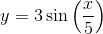?Explanation:

For any equation in the form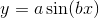, the amplitude of the function is equal to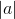.

In this case,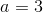and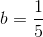, so our amplitude is.

### Example Question #2 : Find The Amplitude Of A Sine Or Cosine Function

What is the amplitude of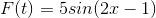?Explanation:

The formula for the amplitude of a sine function isfrom the form: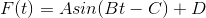.

In our function,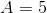.

Therefore, the amplitude for this function is.

### Example Question #3 : Find The Amplitude Of A Sine Or Cosine Function

Find the amplitude of the following trig function: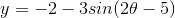Explanation:

Rewriteso that it is in the form of: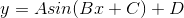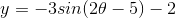The absolute value ofis the value of the amplitude.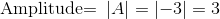### Example Question #4 : Find The Amplitude Of A Sine Or Cosine Function

Find the amplitude of the function.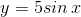Explanation:

For the sine function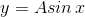where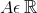the amplitude is given as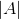.

As such the amplitude for the given functionis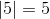.

### Example Question #85 : Functions And Graphs

Which of the given functions has the greatest amplitude?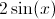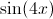The amplitude of a function is the amount by which the graph of the function travels above and below its midline. When graphing a sine function, the value of the amplitude is equivalent to the value of the coefficient of the sine. Similarly, the coefficient associated with the x-value is related to the function's period. The largest coefficient associated with the sine in the provided functions is 2; therefore the correct answer is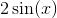.